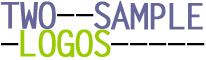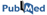Home | Create TSL | Examples | Help

Two Sample Logo is a web-based application that calculates and visualizes differences between two sets of aligned samples of amino acids or nucleotides. Statistical significance is calculated for each residue at each position in the aligned groups of sequences, where the null hypothesis is that the residue is generated according to the same distribution in both positive and negative samples. Two Sample Logos can be used to determine statistically significant residues around various active sites, protein modification sites, or to find differences between two groups of sequences that share the same sequence motif.

The software supports two types of graphical representation: (i) statistically significant residues are plotted using the same size for each residue symbol, (ii) statistically significant symbols are plotted using the size of the symbol that is proportional to the difference between the two samples. Residues are separated in two groups: (i) enriched in the positive sample, and (ii) depleted in the positive sample. In all types of representations, symbols can be plotted using various color schemes and shared residues can be added to the plot in order to visualize the motif itself. The p-value is calculated using the binomial distribution (more accurate, but slower option) or the t-test (less accurate, but significantly faster).

#### Acknowledgements

Two Sample Logo software was developed by Vladimir Vacic (New York Genome Center, New York), Lilia M. Iakoucheva (University of California, San Diego), and Predrag Radivojac (Indiana University, Bloomington).

In citing the Two Sample Logo software, please refer to:

Vacic V., Iakoucheva L.M., and Radivojac P. "Two Sample Logo: A Graphical Representation of the Differences between Two Sets of Sequence Alignments." Bioinformatics, 22(12): 1536-1537. (2006)Two Sample Logo was created using the Ruby programming language, based on the WebLogo code, developed by Gavin E. Crooks, Gary Hon, John-Marc Chandonia and Steven E. Brenner, (Crooks et al., 2004) from the Computational Genomics Research Group, Department of Plant and Microbial Biology, University of California, Berkeley. WebLogo was in turn based on sequence logos developed by Tom Schneider and Mike Stephens (Schneideir and Stephens, 1990). Routines for calculating p-values were written in C and use numerical approximation functions from the Stephen L. Moshier's Cephes Math Library. Web page design for Two Sample Logo was inspired by the WebLogo web interface.